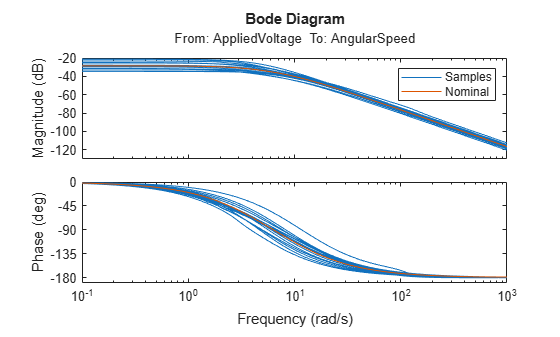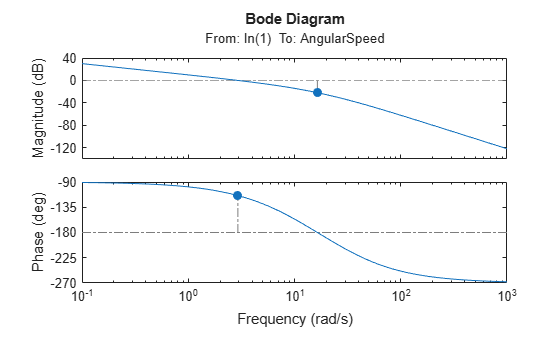## Robustness of Servo Controller for DC Motor

This example shows how to use uncertain objects in Robust Control Toolbox™ to model uncertain systems and to automate robustness calculations using the robustness analysis tools.

### Data Structures for Uncertainty Modeling

Robust Control Toolbox lets you create uncertain elements, such as physical parameters whose values are not known exactly, and combine these elements into uncertain models. You can then easily analyze the impact of uncertainty on the control system performance.

For example, consider a plant model

`$P\left(s\right)=\frac{\gamma }{\tau s+1}$`

where `gamma` can range in the interval [3,5] and `tau` has average value 0.5 with 30% variability. You can create an uncertain model of P(s) as in this example:

```gamma = ureal('gamma',4,'range',[3 5]); tau = ureal('tau',.5,'Percentage',30); P = tf(gamma,[tau 1])```
```P = Uncertain continuous-time state-space model with 1 outputs, 1 inputs, 1 states. The model uncertainty consists of the following blocks: gamma: Uncertain real, nominal = 4, range = [3,5], 1 occurrences tau: Uncertain real, nominal = 0.5, variability = [-30,30]%, 1 occurrences Type "P.NominalValue" to see the nominal value, "get(P)" to see all properties, and "P.Uncertainty" to interact with the uncertain elements. ```

Suppose we have designed an integral controller `C` for the nominal plant (`gamma`=4 and `tau`=0.5). To find out how variations of `gamma` and `tau` affect the plant and the closed-loop performance, we form the closed-loop system `CLP` from `C` and `P`.

```KI = 1/(2*tau.Nominal*gamma.Nominal); C = tf(KI,[1 0]); CLP = feedback(P*C,1)```
```CLP = Uncertain continuous-time state-space model with 1 outputs, 1 inputs, 2 states. The model uncertainty consists of the following blocks: gamma: Uncertain real, nominal = 4, range = [3,5], 1 occurrences tau: Uncertain real, nominal = 0.5, variability = [-30,30]%, 1 occurrences Type "CLP.NominalValue" to see the nominal value, "get(CLP)" to see all properties, and "CLP.Uncertainty" to interact with the uncertain elements. ```

We can now generate 20 random samples of the uncertain parameters `gamma` and `tau` and plot the corresponding step responses of the plant and closed-loop models:

```subplot(2,1,1); step(usample(P,20)), title('Plant response (20 samples)') subplot(2,1,2); step(usample(CLP,20)), title('Closed-loop response (20 samples)')```Figure 1: Step responses of the plant and closed-loop models

The bottom plot shows that the closed-loop system is reasonably robust despite significant fluctuations in the plant DC gain. This is a desirable, and common characteristic of a properly designed feedback system.

### DC Motor Example with Parameter Uncertainty and Unmodeled Dynamics

Now we'll build on the Control System Toolbox™ DC motor example by adding parameter uncertainty and unmodeled dynamics, and investigating the robustness of the servo controller to such uncertainty.

The nominal model of the DC motor is defined by the resistance R, the inductance L, the emf constant Kb, armature constant Km, the linear approximation of viscous friction Kf and the inertial load J. Each of these components varies within a specific range of values. The resistance and inductance constants range within +/- 40% of their nominal values. We use the `ureal` function to construct these uncertain parameters:

```R = ureal('R',2,'Percentage',40); L = ureal('L',0.5,'Percentage',40);```

For physical reasons, the values of Kf and Kb are the same, even if they are uncertain. In this example, the nominal value is 0.015 with a range between 0.012 and 0.019.

```K = ureal('K',0.015,'Range',[0.012 0.019]); Km = K; Kb = K;```

Viscous friction, Kf, has a nominal value of 0.2 with a 50% variation in its value.

`Kf = ureal('Kf',0.2,'Percentage',50);`

### Electrical and Mechanical Equations

The current in the electrical circuit, and the torque applied to the rotor can be expressed in terms of the applied voltage and the angular speed. Create the transfer function `H` relating these variables, and make `AngularSpeed` an output of `H` for later use:

```H = [1;0;Km] * tf(1,[L R]) * [1 -Kb] + [0 0;0 1;0 -Kf]; H.InputName = {'AppliedVoltage';'AngularSpeed'}; H.OutputName = {'Current';'AngularSpeed';'RotorTorque'};```

H is an multi-input, multi-output uncertain system as seen from its display.

`H`
```H = Uncertain continuous-time state-space model with 3 outputs, 2 inputs, 1 states. The model uncertainty consists of the following blocks: K: Uncertain real, nominal = 0.015, range = [0.012,0.019], 2 occurrences Kf: Uncertain real, nominal = 0.2, variability = [-50,50]%, 1 occurrences L: Uncertain real, nominal = 0.5, variability = [-40,40]%, 1 occurrences R: Uncertain real, nominal = 2, variability = [-40,40]%, 1 occurrences Type "H.NominalValue" to see the nominal value, "get(H)" to see all properties, and "H.Uncertainty" to interact with the uncertain elements. ```

The motor typically drives an inertia, whose dynamic characteristics relate the applied torque to the rate-of-change of the angular speed. For a rigid body, this is a constant. A more realistic, but uncertain, model might contain unknown damped resonances. Use the `ultidyn` object to model uncertain linear time-invariant dynamics. The nominal value of the rigid body inertia is set to 0.02 and we add 15% dynamic uncertainty in multiplicative form:

```J = 0.02*(1 + ultidyn('Jlti',[1 1],'Type','GainBounded','Bound',0.15,... 'SampleStateDim',4));```

### Uncertain Model of DC Motor

It is a simple matter to relate the `AngularSpeed` input to the `RotorTorque` output through the uncertain inertia, `J`, using the `lft` command. The AngularSpeed input equals RotorTorque/(J*s), hence "positive" feedback from the 3rd output to the 2nd input of `H` is used to make the connection. This results in a system with 1 input (`AppliedVoltage`) and 2 outputs, (`Current` and `AngularSpeed`).

`Pall = lft(H, tf(1,[1 0])/J);`

Select only the AngularSpeed output for the remainder of the control analysis.

`P = Pall(2,:)`
```P = Uncertain continuous-time state-space model with 1 outputs, 1 inputs, 2 states. The model uncertainty consists of the following blocks: Jlti: Uncertain 1x1 LTI, peak gain = 0.15, 1 occurrences K: Uncertain real, nominal = 0.015, range = [0.012,0.019], 2 occurrences Kf: Uncertain real, nominal = 0.2, variability = [-50,50]%, 1 occurrences L: Uncertain real, nominal = 0.5, variability = [-40,40]%, 1 occurrences R: Uncertain real, nominal = 2, variability = [-40,40]%, 1 occurrences Type "P.NominalValue" to see the nominal value, "get(P)" to see all properties, and "P.Uncertainty" to interact with the uncertain elements. ```

P is a single-input, single-output uncertain model of the DC motor. For analysis purposes, we use the nominal controller synthesized for the DC motor in the Control System Toolbox™ example.

`Cont = tf(84*[.233 1],[.0357 1 0]);`

### Open-Loop Analysis

First, let's compare the step response of the nominal DC motor with 20 samples of the uncertain model of the DC motor:

```clf step(P.NominalValue,'r-+',usample(P,20),'b',3) legend('Nominal','Samples')```Figure 2: Open-loop step response analysis

Similarly, we can compare the Bode plot of the open-loop nominal (red) and sampled (blue) uncertain models of the DC motor.

```om = logspace(-1,2,80); Pg = ufrd(P,om); bode(usample(Pg,25),'b',Pg.NominalValue,'r-+'); legend('Samples','Nominal')```Figure 3: Open-loop Bode plot analysis

### Closed-Loop Robustness Analysis

In this section, we analyze the stability and performance robustness of the closed-loop DC motor system. Our initial analysis of the nominal closed-loop system indicates the nominal closed-loop system is very robust with 10.5 gain margin and 54.3 deg of phase margin.

`margin(P.NominalValue*Cont)`Figure 4: Closed-loop robustness analysis

The `allmargin` and `diskmargin` functions provide comprehensive stability analysis for multivariable feedback systems. For a control system with N feedback channels, the `allmargin` function returns the classical gain and phase margins `SM` for each individual feedback channel (loop-at-a-time margins). The `diskmargin` function returns:

• The disk margins `DM` for each individual feedback channel. The disk margin for the j-th feedback channel indicates by how much the transfer function Lj(s) can vary before this particular loop goes unstable.

• The multi-loop disk margin `MM` This indicates how much simultaneous, independent gain and phase variations can be tolerated in each feedback channel before the overall closed-loop system goes unstable (same as DM for single-loop control systems).

`SM = allmargin(P.NominalValue*Cont)`
```SM = struct with fields: GainMargin: 12.4154 GMFrequency: 16.4229 PhaseMargin: 65.7794 PMFrequency: 2.9349 DelayMargin: 0.3912 DMFrequency: 2.9349 Stable: 1 ```
`[DM,MM] = diskmargin(P.NominalValue*Cont);`

Classical stability margins:

`SM`
```SM = struct with fields: GainMargin: 12.4154 GMFrequency: 16.4229 PhaseMargin: 65.7794 PMFrequency: 2.9349 DelayMargin: 0.3912 DMFrequency: 2.9349 Stable: 1 ```

Disk margin:

`DM`
```DM = struct with fields: GainMargin: [0.2792 3.5822] PhaseMargin: [-58.8054 58.8054] DiskMargin: 1.1271 LowerBound: 1.1271 UpperBound: 1.1271 Frequency: 5.0062 ```

Recall that the DC motor plant is uncertain. In addition to the standard gain and phase margins, we can use the `wcmargin` function to determine the worst-case gain/phase margins for the plant-controller feedback loop. The `wcmargin` function calculates the worst-case disk gain and phase margins for each input/output channel. The worst-case analysis shows a possible degradation of the disk gain and phase margins, which were 11 dB and 59 degs respectively, to 1.2dB and 8 degs, respectively, in the presence of the 5 forms of uncertainty modeled in `P`.

`wcmarg = wcmargin(Pg,Cont)`
```wcmarg = struct with fields: GainMargin: [0.8709 1.1482] PhaseMargin: [-7.8917 7.8917] Frequency: 5.1152 WCUnc: [1x1 struct] Sensitivity: [1x1 struct] ```

### Robustness of Disturbance Rejection Characteristics

The sensitivity function is a standard measure of closed-loop performance for the feedback system. Let's compute the uncertain sensitivity function `S` and compare the Bode magnitude plots for the nominal and sampled uncertain sensitivity function.

```S = feedback(1,P*Cont); bodemag(usample(S,20),'b',S.Nominal,'r-+'); legend('Samples','Nominal')```Figure 5: Magnitude of sensitivity function S.

In the time domain, the sensitivity function indicates how well a step disturbance can be rejected. Let's sample the uncertain sensitivity function and plot its step response to see the variability in disturbance rejection characteristics (nominal appears in red).

```step(usample(S,20),'b',S.Nominal,'r-+',3); title('Disturbance Rejection') legend('Samples','Nominal')```Figure 6: Rejection of a step disturbance.

Use the `wcgain` function to compute the worst-case value of the uncertain sensitivity function gain (peak across frequency).

```Sg = ufrd(S,om); [maxgain,worstuncertainty] = wcgain(Sg); maxgain```
```maxgain = struct with fields: LowerBound: 7.4366 UpperBound: 7.4487 CriticalFrequency: 5.1152 ```

With the `usubs` function you can substitute the worst-case values of the uncertainty `worstuncertainty` into the uncertain sensitivity function `S`. This gives the worst-case sensitivity function `Sworst` over the entire uncertainty range. Note that the peak gain of `Sworst` matches the lower-bound computed by `wcgain`.

```Sworst = usubs(S,worstuncertainty); Sgworst = frd(Sworst,Sg.Frequency); norm(Sgworst,inf)```
```ans = 7.4366 ```
`maxgain.LowerBound`
```ans = 7.4366 ```

Now let's compare the step responses of the nominal and worst-case sensitivity:

```step(Sworst,'b',S.NominalValue,'r-+',6); title('Disturbance Rejection') legend('Worst-case','Nominal')```Figure 7: Nominal and worst-case rejection of a step disturbance

Finally, let's plot Bode magnitude plots of the nominal and worst-case values of the sensitivity function. Observe that the peak value of `Sworst` occurs at the frequency `maxgain.CriticalFrequency`:

```bodemag(Sg.NominalValue,'r-+',Sgworst,'b'); legend('Worst-case','Nominal') hold on semilogx(maxgain.CriticalFrequency,20*log10(maxgain.LowerBound),'g*') hold off```Figure 8: Magnitude of nominal and worst-case sensitivity

##### SupportGet trial now